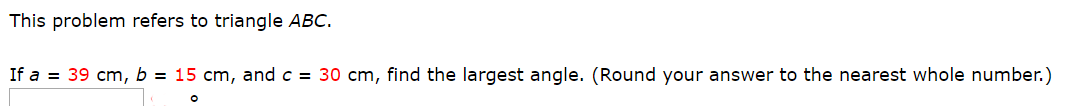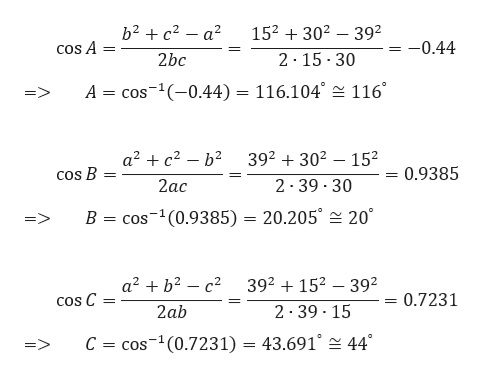# This problem refers to triangle ABC.If a39 cm, b = 15 cm, and c = 30 cm, find the largest angle. (Round your answer to the nearest whole number.)

Question
8 views

Photo attachedhelp_outlineImage TranscriptioncloseThis problem refers to triangle ABC. If a 39 cm, b = 15 cm, and c = 30 cm, find the largest angle. (Round your answer to the nearest whole number.) fullscreen
check_circle

star
star
star
star
star
1 Rating
Step 1

Let’s first draw the given triangle ABC for better understanding. Here a = 39,

b = 15 and c = 30.

Step 2

Using Cosine rule for a trian...help_outlineImage Transcriptioncloseb2 c2 a2 152 302 392 -0.44 cos A 2 15 30 2bc cos (-0.44) = 116.104° 116° А: => a2c2b2 392 302 152 0.9385 COS B 2ас 2 39 30 20 B cos-(0.9385) = 20.205 => a2b2 c2 392152 392 0.7231 COS C 2 39 15 2ab C = cos-1(0.7231) = 43.691° 44 => fullscreen

### Want to see the full answer?

See Solution

#### Want to see this answer and more?

Solutions are written by subject experts who are available 24/7. Questions are typically answered within 1 hour.*

See Solution
*Response times may vary by subject and question.
Tagged in

### Trigonometry